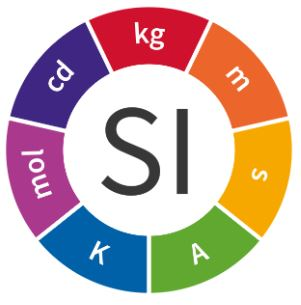# Units

A unit of measurement is a definite magnitude of a quantity, defined and adopted by convention or by law, that is used as a standard for measurement of the same quantity. Any other value of that quantity can be expressed as a simple multiple of the unit of measurement. For example, length is a physical quantity.description value detail
None none No applicable units.
Megatonnes or million tonnes per annum Mtpa Megatonnes or million tonnes per annum
Voltage V Voltage, electric potential difference, electric pressure or electric tension (formally denoted ∆V or ∆U, but more often simply as V or U, for instanc...
Centigrade °C Celsius, also known as centigrade, is a metric scale and unit of measurement for temperature. As an SI derived unit, it is used by most countries in t...
Metre m The metre (international spelling) or meter (American spelling), (from the Greek noun μέτρον, "measure") is the base unit of length in the Internation...
Hertz Hz The hertz (symbol Hz) is the unit of frequency in the International System of Units (SI) and is defined as one cycle per second.
URL url A Uniform Resource Locator (URL), colloquially termed a web address, is a reference to a web resource that specifies its location on a computer networ...
modulus of elasticity kN/m The modulus of elasticity (also known as the elastic modulus, the tensile modulus, or Young's modulus) is a number that measures an object or substanc...
millimetre mm The millimetre (International spelling as used by the International Bureau of Weights and Measures; SI unit symbol mm) or millimeter (American spellin...
percentage % In mathematics, a percentage is a number or ratio expressed as a fraction of 100. It is often denoted using the percent sign, "%", or the abbreviation...
density kg/m³ Kilogram per cubic metre is an SI derived unit of density, defined by mass in kilograms divided by volume in cubic metres. The official SI symbolic ab...
Force kN The newton is the International System of Units derived unit of force. It is named after Isaac Newton in recognition of his work on classical mechanic...
Revolutions per minute r/min Revolutions per minute (abbreviated rpm, RPM, rev/min, r/min) is a measure of the frequency of rotation, specifically the number of rotations around a...
Kilograms per meter Kg/m Kilograms per meter
kilogram Kg The kilogram (abbreviation, kg) is the Standard International (SI) System of Units unit of mass. It is defined as the mass of a particular internation...
kilogram per square metre kg/m² kilogram per square metre
Degree Degree A degree (in full, a degree of arc, arc degree, or arcdegree), usually denoted by ° (the degree symbol), is a measurement of a plane angle, defined so...
Metres per second. m/s m/s is the abbreviation for metre per second.
Tonnes per hour TPH Measure of tonnes per hour.
kilopascal kPa The pascal (symbol: Pa) is the SI derived unit of pressure used to quantify internal pressure, stress, Young's modulus and ultimate tensile strength. ...
Joule J The joule (/dʒuːl/); symbol: J), is a derived unit of energy in the International System of Units. It is equal to the energy transferred to (or wor...
Kilowatt kW Kilowatt (symbol: kW) is a unit of electric power.
Ampere A a unit of electric current equal to a flow of one coulomb per second.
Ampere kA a unit of electric current equal to a flow of one coulomb per second.
Megapascal MPa The pascal (symbol: Pa) is the SI derived unit of pressure used to quantify internal pressure, stress, Young's modulus and ultimate tensile strength. ...
meters per minute. m/min meters per minute.
Tonne tonne The tonne (non-preferred SI derived unit; SI symbol: t), commonly referred to as the metric ton in the United States, is a non-SI metric unit of mass ...
Newton metre N m The newton metre (also newton-metre, symbol N m or N⋅m) is a unit of torque (also called "moment") in the SI system. One newton metre is equal to t...
Inertia kg. m² Inertia is the resistance, of any physical object, to any change in its velocity. This includes changes to the object's speed, or direction of motion.
Minute and second of arc arcmin A minute of arc, arcminute (arcmin), arc minute, or minute arc is a unit of angular measurement
Hour Hr An hour (symbol: h; also abbreviated hr.) is a unit of time
Second s The second (symbol: s) is the base unit of time in the International System of Units (SI), commonly understood and historically defined as ​1⁄86400 of...
PIW piw Piw means pounds per inch of belt width and is widely used in the United States of America. The piw figure is indicating the working tension a conveyo...

#### Collaborate

Would you like to list your product or become a local Shiftbulk agent?

its free.

#### Issues

List your issues with the interface.

#### More details

Terms | Privacy | Contact

###Shiftbulk

Shiftbulk is a collaborative platform for creating detailed design calculations and purchasing schedules for belt conveyor systems

Built with Heavy-lifting and Fraternate